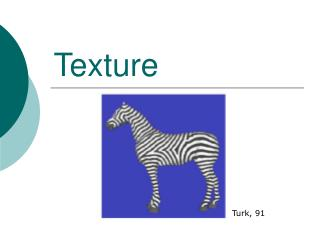DownloadDownload PresentationTexture

# Texture

Télécharger la présentation## Texture

- - - - - - - - - - - - - - - - - - - - - - - - - - - E N D - - - - - - - - - - - - - - - - - - - - - - - - - - -
##### Presentation Transcript

1. Texture Turk, 91

2. What is texture ? • There is no accurate definition. • It is often used to represent all the “details” in the image. (F.e, sometimes images are divided to shape + texture. • In our case we refer to the texture as images or patterns with some kind of “structure”.

3. What is texture ? (cont’) repetition stochastic both fractal

4. What would we like to do with textures? • Detect regions / images with textures. • Classify using texture. • Segmentation: divide the image into regions with uniform texture. • Synthesis – given a sample of the texture, generate random images with the same texture. • Compression (Especially fractals) Why is it difficult ?

5. Periodic Texture • big assumption: the image is periodic, completely specified by a fundamental region. • no allowance for statistical variations • this approach is fine if image is periodic, but too limited as a general texture model.

6. Texture primitives • The basic elements that form the texture. • Sometimes, they are called “texels”. • Primitives do not always exists ( or are not visible). • In textures which are not periodic, the texel is the “essential” size of the texture. • There might be textures with structure in several resolutions (bricks) • Fractals have the similar structure in each resolution. “we don’t see the forest.”

7. Texture Description • Auto-correlation • Fourier Transform in small windows • Wavlets or Filter banks • Feature vectors • Statistical descriptors • Markov Chains • …

8. The auto-correlation • Describes the relations between neighboring pixels. • Equivalently, we can analyze the power spectrum of the window: We apply a Fourier Transform in small windows. • Analyzing the power spectrum: • Periodicity: The energy of different frequencies. • Directionality: The energy of slices in different directions.

9. Filter banks • Instead of using the Fourier Basis, apply filters which best classify different textures. • Use filters of varying orientations. • Use filters of varying scales: • Laplacian pyramids • Wavlets pyramids • … • Gabor Filters (Local sinuses in varying scales and directions). • Filters which describe certain properties ( Entropy, Energy, Coarseness…) • Some successful results in texture segmentation were achieved using moment-based features (mean, variance)

10. What can we do with these features? • For each pixel (or window) attach a vector of features. • Use this vector to calculate the “distance” between different windows. • We can compute statistics of the features in a region: • Use the statistics to separate between different textures. • … • We can determine the “essential” size of the texture: the size in which the statistics are “interesting”.

11. Second order statistics (or co-occurrence matrices) • The intensity histogram is very limited in describing a texture (f.e - checkerboard versus white-black regions. • Use higher-level statistics: Pairs distribution. • From this matrix, generate a list of features: • Energy • Entropy (can also be used as a measure for “textureness”). • Homogeneity ( ) 0 1 2 3 • Example: • co-occurrence matrix of I(x,y) and I(x+1,y) • Normalize the matrix to get probabilities. 0 1 2 3

12. Texture as a Stochastic Process • A random variable is a value with a given probability distribution. • A discrete stochastic process is a sequence or array of random variables, statistically interrelated. • Conditional probability P[A|B,C] means probability of A given B and C

13. Markov Chain • Assume that each variable depends only on the n preceding values. • In this case, we have a Markov chain of order n. • We estimate the process using an histogram of groups of size n (The co-occurrence matrix is a special case with n=2) • We can use this process to synthesis new images ! • Markov Random Field: The same, but 2D.

14. Markov Chain Example Output of 2nd order word-level Markov Chain [Scientific American, June 1989, Dewdney] after training on 90,000 word philosophical “If we were to revive the fable is useless. Perhaps only the allegory of simulation is unendurable--more cruel than Artaud’s Theatre of Cruelty, which was the first to practice deterrence, abstraction, disconnection, deterritorialisation, etc.; and if it were our own past. We are witnessing the end of the negative form. But nothing separates one pole from the very swing of voting ’’rights’’ to electoral...”

15. Region texels • Divide the image into uniform regions. • Use this regions as the texels, or image primitives. • Use the structure of this regions to make a statistics about the texture. For example: • Directionality • diameter versus boundary length

16. Shape from texture • Under the assumption of isotropic patterns, we can use this to recover shape. • If the texture is periodic, we can use the size differences between the primitives to recover shape. • For example, assuming a planar scene, we can use the direction of maximum rate of change of the primitives size: “texture gradient”

17. Summery • There are many ways to describe a texture: • Different kinds of filters. • Statistical descriptors. • Texture as a random process. • For each pixel/region we attach the vector of features. • Some works try to recover the primitives. In some cases, it can be used to learn the 3D shape. • Many applications. • For example: Texture synthesis.